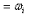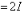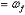×
Get Full Access to Physics: Principles With Applications - 6 Edition - Chapter 8 - Problem 59p
Get Full Access to Physics: Principles With Applications - 6 Edition - Chapter 8 - Problem 59p

×

# (II) A nonrotating cylindrical disk of moment of inertia IISBN: 9780130606204 3

## Solution for problem 59P Chapter 8

Physics: Principles with Applications | 6th Edition

• Textbook Solutions
• 2901 Step-by-step solutions solved by professors and subject experts
• Get 24/7 help from StudySoup virtual teaching assistantsPhysics: Principles with Applications | 6th Edition

4 5 1 359 Reviews
17
5
Problem 59P

(II) A nonrotating cylindrical disk of moment of inertia I is dropped onto an identical disk rotating at angular speed co. Assuming no external torques, what is the final common angular speed of the two disks?

Step-by-Step Solution:

Step 1 of 2

The cylindrical disk of the moment of inertia I is rotating with an angular speedThen a non-rotating cylindrical disk of the same moment of inertia I is dropped on the rotating cylinder.

As the net torque = 0, hence the angular momentum is conserved.

The coupled system now has rotational inertiaAnd let the final angular speed of this coupled system beStep 2 of 2

##### ISBN: 9780130606204

Physics: Principles with Applications was written by and is associated to the ISBN: 9780130606204. Since the solution to 59P from 8 chapter was answered, more than 705 students have viewed the full step-by-step answer. This full solution covers the following key subjects: angular, speed, disk, final, common. This expansive textbook survival guide covers 35 chapters, and 3914 solutions. This textbook survival guide was created for the textbook: Physics: Principles with Applications, edition: 6. The answer to “?(II) A nonrotating cylindrical disk of moment of inertia I is dropped onto an identical disk rotating at angular speed co. Assuming no external torques, what is the final common angular speed of the two disks?” is broken down into a number of easy to follow steps, and 36 words. The full step-by-step solution to problem: 59P from chapter: 8 was answered by , our top Physics solution expert on 03/03/17, 03:53PM.

## Discover and learn what students are asking

Statistics: Informed Decisions Using Data : Tests for Independence and the Homogeneity of Proportions
?Profile of Smokers The following data represent the smoking status from a random sample of 1054 U.S. residents 18 years or older by level of education

Unlock Textbook Solution

Enter your email below to unlock your verified solution to:

(II) A nonrotating cylindrical disk of moment of inertia I